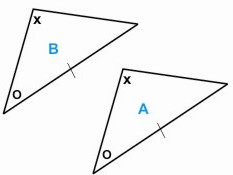HOME MATH DICTIONARY DOWNLOAD FEEDBACK DISCLAIMER
 Question: What is Component ? Answer:The displacement shown in the diagram has a component of 3 in the x-direction and a component of 4 in the y-direction. It could be written as a column vector. 3 and 4 are called the components of the vector. In elementary mathematics and physics, a vector is a geometric object that has both a magnitude (or length) and a direction. A vector is graphically represented by an arrow. The magnitude of the vector is proportional to the length of the arrow and its direction coincides with the direction of the arrow.